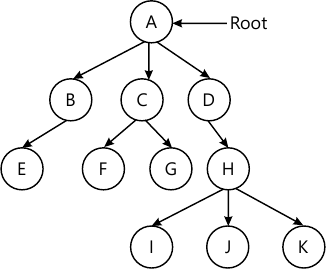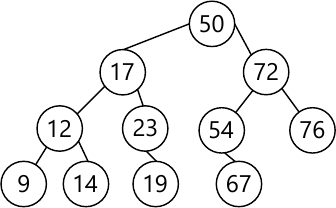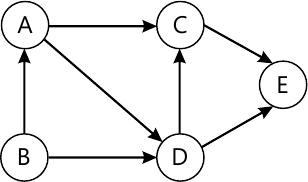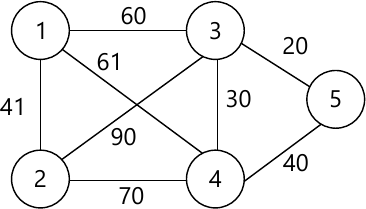MORE IN Data Structures Algorithm
SPPU Computer Engineering (Semester 3)
Data Structures Algorithm
May 2014
Total marks: --
Total time: --
INSTRUCTIONS
(1) Assume appropriate data and state your reasons
(2) Marks are given to the right of every question
(3) Draw neat diagrams wherever necessary

Answer any one question from Q1 and Q2
1 (a) Find the frequency count for the following code.
for(i=1;i<=n;i++)
{
for(i=1;i<=n;i++)
{
for(i=1;i<=n;i++)
{
sum=sum+I;
}
}
}
3 M
1 (b) Write a function for selection sort using templates.
3 M
6 M

2 (a) Explain different Asymptotic notations.
3 M
2 (b) Convert the following tree into Binary tree.6 M
2 (c) Consider the following tree given in the problem. Show a Postorder, Preorder and In order Traversal of the tree.3 M

Answer any one question from Q3 and Q4
3 (a) Sort the digraph for topological sort refer figure 1 below.3 M
3 (b) Convert given graph into MST refer figure 2 below.3 M
3 (c) What is collision? What Are different collision resolution techniques?
3 M
3 (d) Draw a binary search tree for the following data 10, 08, 15, 12, 13, 07, 09, 17, 20, 18, 04, 05.
3 M

4 (a) Explain with suitable example the various storage structures for the graph.
6 M
4 (b) Construct the AVL tree for the following data by inserting each data item one at a time. 15, 20, 24, 10, 13, 7, 30, 36, 25.
6 M

Answer any one question from Q5 and Q6
5 (a) Sort the following data in ascending order using heap sort.15, 19, 10, 7, 17, 16.
6 M
5 (b) Write an algorithm to search an element in a B Tree.
4 M
5 (c) Define sequential file organization and state its advantages and disadvantages.
3 M

6 (a) What is ISMA in file organization? Explain Advantages & Disadvantages of sequential file organization.
6 M
6 (b) What is a B+ tree? Give structure of its internal node. What are the order of B+ tree & Characteristics of B+ tree.
7 M

Answer any one question from Q7 and Q8
7 (a) Explain in detail the models used for parallel computation.
6 M
7 (b) Write a parallel algorithm to perform the addition of the given numbers using complete binary tree method.
7 M

8 (a) Write a parallel algorithm for pointer doubling. Explain with suitable example.
7 M
8 (b) Write a parallel algorithm for odd-even merge sort.
6 M

More question papers from Data Structures Algorithm